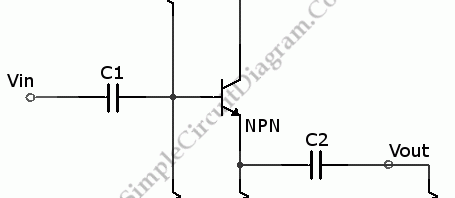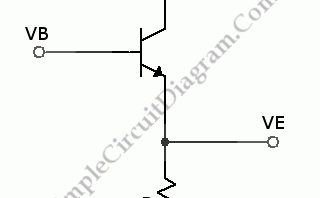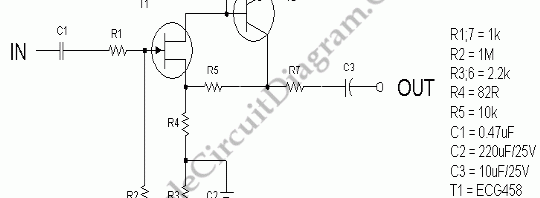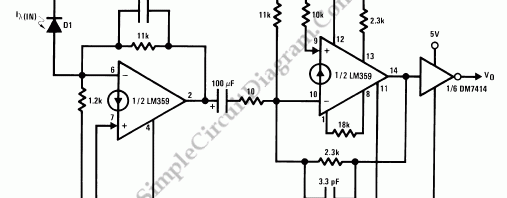## NPN Transistor Common Collector AmplifierThis is circuit of NPN Common Collector Amplifier. This circuit can be used for impedance matching. This circuit similar to the Emitter Follower circuit because the emitter of this circuit is follows the base minus a diode drop. This circuit has voltage gain of one because the output voltage is forced to follow about 0.6V below the signal input. Here […]

## Transistor Amplifier – Emitter FollowerThis a Emitter Follower circuit. This circuit is also called The common collector junction transistor amplifier. This amplifier has little voltage gain, less than one, because the emitter voltage is forced to drop about 0.6V below the base. This circuit is used for impedance matching and power or current gain. Here is the schematic diagram of the circuit: The signal […]

## JFET Low Noise PreampNoise, in amplifier circuit, is generated intrinsically by each components inside the circuit. Resistor, capacitor, and semiconductor generate noises. By simplifying the design of a circuit, we can minimize the noise especially if we can push the number of components to the lowest count. Here is one example of such design. The gain of this low noise amplifier is determined […]